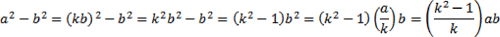SEARCH HOMEMath Central Quandaries & QueriesQuestion from Nazrul, a teacher: If a is proportional to b then how can I prove that (a^2-b^2) is proportional to ab. Please show me the process in details.If a is proportional to b then a = kb for some constant k. (lets consider only a,b,k nonzero).

Then a2-b2 = (kb)2 - b2 = (k2-1)b2

and ab = (kb)b=kb2.

Thus (a2 - b2) / ab = (k2-1)b2/(kb2) = (k2-1)/k which is a constant,
i.e. a2 - b2 = ab * (k2-1)/k, i.e. a2-b2 is equal to ab times a constant.

Thus if a is proportional to b then a2-b2 is proportional to ab (for b not equal to zero).

Thx,
ms. Dame

Hello Nazrul,
If ‘a’ is proportional to ‘b’ then  a = kb, for some number ‘k’.  So,Let m = (k2 - 1)/k then a2 - b2 = m ab.

Thus a2 - b2 is proportional to ab.

TylerMath Central is supported by the University of Regina and The Pacific Institute for the Mathematical Sciences.